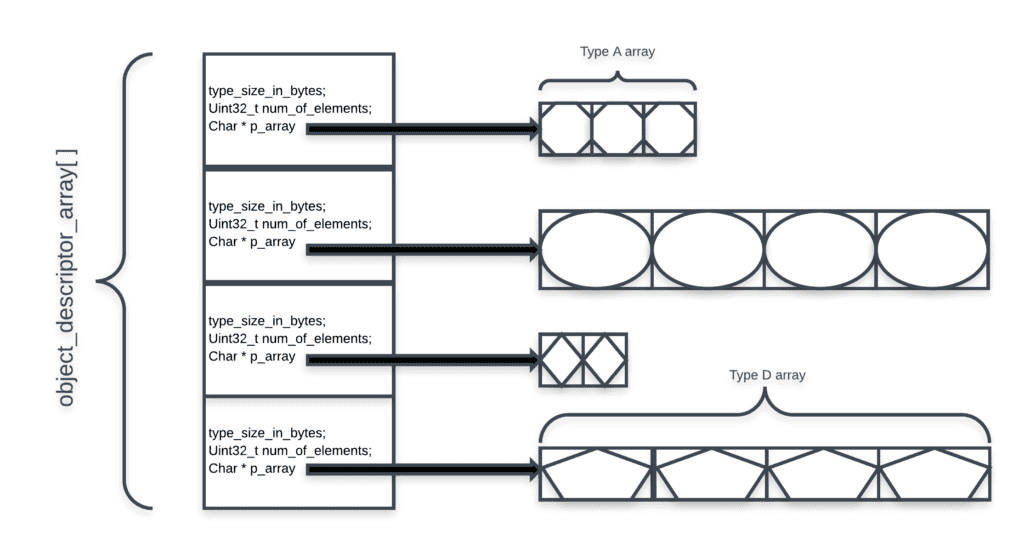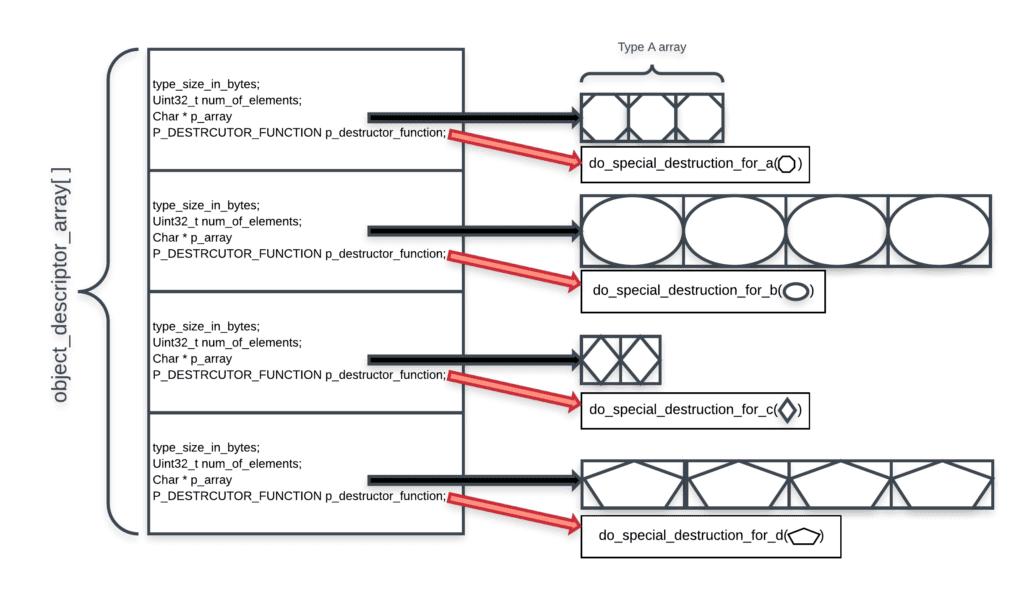# C Polymorphism – Part 2

In the first part of this post, a “classic” example of Polymorphism-like code in C was discussed. Let’s look at some production code I had a chance to refactor. After the refctoring, the code looks like another step forward towards the Polymorphic behavior of real OOP languages. For obvious reasons, I have modified the example such that it is more focused.

The original code used an array of object types. For each one, an array of instances was allocated. The “Type structure” looked like that:

typedef struct

{

unsigned int type_size_in_bytes;

unsigned int num_of_elements;

// This will point to the allocated array of instances

char * p_array;

}TYPE_DESCRIPTOR;

And, there was an array of those “descriptor” objects plus an enum to enumerate the different types:

typedef enum

{

OBJECT_TYPE_A = 0,

OBJECT_TYPE_B,

// And so on, types C, D, E, etc

OBJECT_TYPE_Z,

//This must not used as a type,

//it simply signifies how many types we have

NUM_OF_TYPES

}OBJECT_TYPES;

TYPE_DESCRIPTOR object_descriptor_array[NUM_OF_TYPES];

So, this is a visualization of how this entire thing looked like if NUM_OF_TYPES equals 4:At times, that entire data structure had to be destroyed and freed. Each object had to be gracefully destroyed by using a function a function that specializes in destroying that specific type. Below are the prototypes of the functions for destroying types A and B. You can see that each function also receives a pointer to the specific type as an argument, so every destroyer function is different.

void destroy_type_a(TYPE_A * p_element);

void destroy_type_b(TYPE_B * p_element);

You can figure out the rest (about a dozen of them).
In order to destroy the entire data structure, a for loop is used that iterates over the type descriptors array, then decends into each descriptor’s element array, iterating over it and destroying each element:

unsigned int object_type;

for(object_type = 0;

object_type > NUM_ELEMENTS(object_descriptor_array);

++object_type)

{

unsigned int current_type_size_bytes =

object_descriptor_array[object_type].type_size_in_bytes;

unsigned int num_of_elements_for_current_type =

object_descriptor_array[object_type].num_of_elements;

unsigned int p_current_type_elements_array =

object_descriptor_array[object_type].p_array;

char * current_element_index;

for(current_element_index = 0;

current_element_index < num_of_elements_for_current_type;

++current_element_index)

{

unsigned int location = current_element_index *                                                          current_type_size_bytes;

void * p_current_element = p_current_type_elements_array[location];

//Based on the numeric order of the object,

//decide which destroyer function to call

if(OBJECT_TYPE_A == object_type)

{

destroy_type_a( (TYPE_A_t*)p_current_element );

}

else if(OBJECT_TYPE_B == object_type)

{

destroy_type_b( (TYPE_B_t*)p_current_element );

}

// And so on, types C, D, E, etc

else if(OBJECT_TYPE_Z == object_type)

{

destroy_type_z((TYPE_Z_t*)p_current_element );

}

}

}

## A Polymorphic version of the same code

Using the following transformation, we can create general code that frees the objects without “manual” if-else clauses:
TYPE_DESCRIPTOR will contain a pointer to a common function pointer prototype. Each TYPE_DESCRIPTOR instance’s pointer will be initialized with the proper destructor function. The destruction loop will call the pointed function relevant to that type.

First, we need to define the prototype for ‘a’ destructor function:

typedef void (*P_DESTRUCTOR_FUNCTION)(void * p_element);

Using this general prototype, we can create the specific destructor functions, each dealing with different data structure and logic:

void destroy_type_a(void * p_element)

{

TYPE_A_t * p_a_element = (TYPE_A_t*)p_element;

do_special_destruction_for_a(p_a_element); //This
function can do whatever is needed to destroy an ‘A’ element

}

void destroy_type_b(void * p_element)

{

TYPE_B_t * p_b_element = (TYPE_B_t*)p_element;

do_special_destruction_for_b(p_b_element); //This
function can do whatever is needed to destroy a ‘B’ element

}

And so on.
Now we can re-define TYPE_DESCRIPTOR:

typedef struct

{

unsigned int type_size_in_bytes;

unsigned int num_of_elements;

char * p_array; // This will point to the allocated array of instances

P_DESTRUCTOR_FUNCTION  p_destructor_function;

}TYPE_DESCRIPTOR;

So now, our data structure looks more like this:And finally, our loop collapses to be just that:

int object_type;

for(object_type = 0;

object_type < NUM_ELEMENTS(object_descriptor_array);

++object_type)

{

unsigned int current_type_size_bytes =

object_descriptor_array[object_type].type_size_in_bytes;

unsigned int num_of_elements_for_current_type =

object_descriptor_array[object_type].num_of_elements;

unsigned int p_current_type_elements_array =

object_descriptor_array[object_type].p_array;

P_DESTRUCTOR_FUNCTION p_destructor_function =

object_descriptor_array[object_type].p_destructor_function;

char * current_element_index;

for(current_element_index = 0;

current_element_index < num_of_elements_for_current_type;

++current_element_index)

{

unsigned int location = current_element_index *                                                          current_type_size_bytes;

void * p_current_element = p_current_type_elements_array[location];

p_destructor_function(p_current_element);

}

}

## Pros and Cons

### Pros

The Polimorphic version of the code is obviously more elegant and removes a lot of code repetition. The original code contains a hidden dependency – the order enum defining the order of the objects, and initialization order of the object_descriptor_array, must be the same! Imagine someone do this one day:

typedef enum

{

OBJECT_TYPE_B = 0, // Type B is now first

OBJECT_TYPE_A, // Type A is now second

// And so on, types C, D, E, etc

OBJECT_TYPE_Z,

//This must not used as a type,

//it simply signifies how many types we have

NUM_OF_TYPES

}OBJECT_TYPES;

Without changing the initialization of object_descriptor_array appropriately, the code is broken. It will match the OBJECT_TYPE_A destructor with OBJECT_TYPE_B instances, and vice versa.
This cannot happen in the new version of the code

### Cons(?)

Generally speaking, code that involves pointers to functions may suffer from the side effects like:

1. Harder to follow and predict since functionality can change on the go.
2. Harder to debug, as you can do less by just reading the code.
3. Some static analysis may not be possible (e.g. Stack usage analysis)

So I think it boils down to good measure, like in many cases. In the example above, I think the gain worth the cost. The usage of function pointers is contained, allows several benefits.

## Further Steps

Potentially, this can be taken to the next level in which each instance has its own function pointer. It is not possible to have a C array with different element sizes, but if for example a linked list is used, a next level of Polymorphism can be achieved. In general, it starts to feel like closing in on how an OOP “under the hood” implementation may look like. Is it recommended? Not necessarily. It gets more and more complex, and you have to weigh in that comlexity against what you gain. You may end up with something harder to maintain and debug than the code you started with. If you find yourself in that position, you may want to consider C++…